Skip to main content

# Existence of Positive Solution to Second-Order Three-Point BVPs on Time Scales

## Abstract

We are concerned with the following nonlinear second-order three-point boundary value problem on time scales,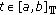,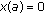,, where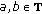with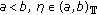and. A new representation of Green's function for the corresponding linear boundary value problem is obtained and some existence criteria of at least one positive solution for the above nonlinear boundary value problem are established by using the iterative method.

## 1. Introduction

Letbe a time scale, that is,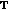is an arbitrary nonempty closed subset of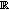. For each intervalof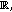we define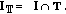For more details on time scales, one can refer to . Recently, three-point boundary value problems (BVPs for short) for second-order dynamic equations on time scales have received much attention. For example, in 2002, Anderson  studied the following second-order three-point BVP on time scales: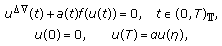(11)

where,,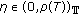and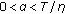. Some existence results of at least one positive solution and of at least three positive solutions were established by using the well-known Krasnoselskii and Leggett-Williams fixed point theorems. In 2003, Kaufmann  applied the Krasnoselskii fixed point theorem to obtain the existence of multiple positive solutions to the BVP (1.1). For some other related results, one can refer to  and references therein.

In this paper, we are concerned with the existence of at least one positive solution for the following second-order three-point BVP on time scales: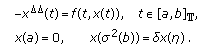(12)

Throughout this paper, we always assume that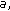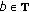with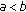,, and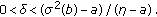It is interesting that the method used in this paper is completely different from that in [6, 7, 9, 10], that is, a new representation of Green's function for the corresponding linear BVP is obtained and some existence criteria of at least one positive solution to the BVP (1.2) are established by using the iterative method.

For the function, we impose the following hypotheses:

(H1)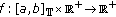is continuous;

(H2)for fixed,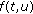is monotone increasing on;

(H3)there exists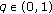such that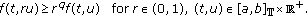(13)

Remark 1.1.

If (H3) is satisfied, then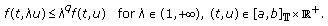(14)

## 2. Main Results

Lemma 2.1.

The BVP (1.2) is equivalent to the integral equation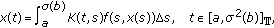(21)

where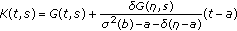(22)

is called the Green's function for the corresponding linear BVP, here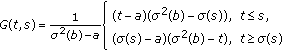(23)

is the Green's function for the BVP: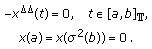(24)

Proof.

Let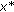be a solution of the BVP: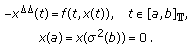(25)

Then, it is easy to know that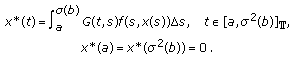(26)

Now, ifis a solution of the BVP (1.2), then it can be expressed by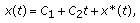(27)

which together with the boundary conditions in (1.2) and (2.6) implies that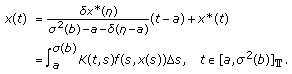(28)

On the other hand, ifsatisfies (2.1), then it is easy to verify thatis a solution of the BVP (1.2).

Lemma 2.2.

For any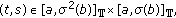one has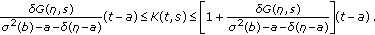(29)

Proof.

Since it is obvious from the expression of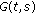that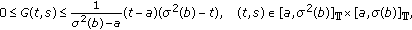(210)

we know that (2.9) is fulfilled.

Our main result is the following theorem.

Theorem 2.3.

Assume that (H1)–(H3) are satisfied. Then, the BVP (1.2) has at least one positive solution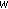. Furthermore, there exist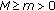such that(211)

Proof.

Let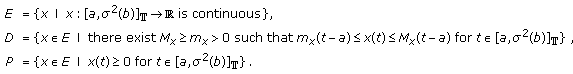(212)

Define an operator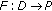: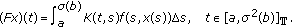(213)

Then it is obvious that fixed points of the operator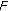inare positive solutions of the BVP (1.2).

First, in view of (H2), it is easy to know thatis increasing.

Next, we may assert that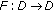, which implies that for any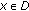, there exist positive constantsandsuch that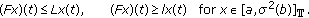(214)

In fact, for any, there exist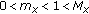such that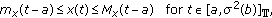(215)

which together with (H2), (H3), and Remark 1.1 implies that(216)

By Lemma 2.2 and (2.16), for any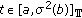, we have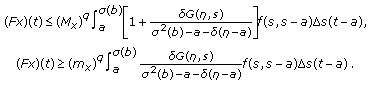(217)

If we let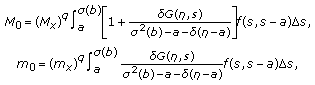(218)

then it follows from (2.17) and (2.18) that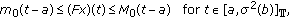(219)

which shows that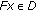.

Now, for any fixed, we denote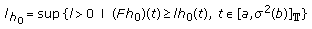(220)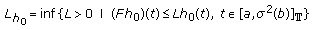(221)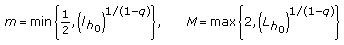(222)

and let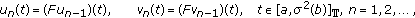(223)

where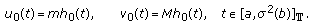(224)

Then, it is easy to know from (2.20), (2.21), (2.22), (2.23), (2.24), (H3), and Remark 1.1 that(225)

Moreover, if we let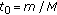, then it follows from (2.22), (2.23), (2.24), and (H3) by induction that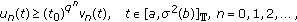(226)

which together with (2.25) implies that for any positive integersand,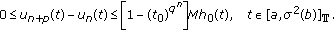(227)

Therefore, there exists asuch that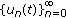and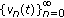converge uniformly toon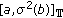and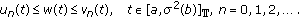(228)

Sinceis increasing, in view of (2.28), we have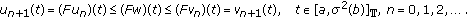(229)

So,(230)

which shows thatis a positive solution of the BVP (1.2). Furthermore, since, there existsuch that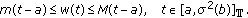(231)

## References

1. 1.

Agarwal RP, Bohner M: Basic calculus on time scales and some of its applications. Results in Mathematics 1999, 35(1–2):3–22.

2. 2.

Bohner M, Peterson A: Dynamic Equations on Time Scales: An Introduction with Application. Birkhäuser, Boston, Mass, USA; 2001:x+358.

3. 3.

Bohner M, Peterson A: Advances in Dynamic Equations on Time Scales. Birkhäuser, Boston, Mass, USA; 2003:xii+348.

4. 4.

Hilger S: Analysis on measure chains—a unified approach to continuous and discrete calculus. Results in Mathematics 1990, 18(1–2):18–56.

5. 5.

Lakshmikantham V, Sivasundaram S, Kaymakcalan B: Dynamic Systems on Measure Chains, Mathematics and Its Applications. Volume 370. Kluwer Academic Publishers, Dordrecht, The Netherlands; 1996:x+285.

6. 6.

Anderson DR: Solutions to second-order three-point problems on time scales. Journal of Difference Equations and Applications 2002, 8(8):673–688. 10.1080/1023619021000000717

7. 7.

Kaufmann ER: Positive solutions of a three-point boundary-value problem on a time scale. Electronic Journal of Differential Equations 2003, 2003(82):-11.

8. 8.

Khan RA, Nieto JJ, Otero-Espinar V: Existence and approximation of solution of three-point boundary value problems on time scales. Journal of Difference Equations and Applications 2008, 14(7):723–736. 10.1080/10236190701840906

9. 9.

Luo H, Ma Q: Positive solutions to a generalized second-order three-point boundary-value problem on time scales. Electronic Journal of Differential Equations 2005, 2005(17):-14.

10. 10.

Sun H-R, Li W-T: Positive solutions for nonlinear three-point boundary value problems on time scales. Journal of Mathematical Analysis and Applications 2004, 299(2):508–524. 10.1016/j.jmaa.2004.03.079

Download references

## Acknowledgment

This work is supported by the National Natural Science Foundation of China (10801068).

## Author information

Authors

### Corresponding author

Correspondence to Jian-Ping Sun.

## Rights and permissions

Open Access This article is distributed under the terms of the Creative Commons Attribution 2.0 International License (https://creativecommons.org/licenses/by/2.0), which permits unrestricted use, distribution, and reproduction in any medium, provided the original work is properly cited.

Reprints and Permissions

## About this article

### Cite this article

Sun, JP. Existence of Positive Solution to Second-Order Three-Point BVPs on Time Scales. Bound Value Probl 2009, 685040 (2009). https://doi.org/10.1155/2009/685040

Download citation

• Received:

• Accepted:

• Published:

### Keywords

• Positive Integer
• Integral Equation
• Partial Differential Equation
• Ordinary Differential Equation
• Functional Equation